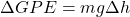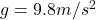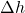Question

a large drill is used to make a hole through rocks in the earths surface to excavate for oil
what’s the equation that links change in gradational potential energy store , mass , gravitational field strength ,height

1.Explanation:

The gravitational potential energy of an object is the energy possessed by the object due to its location in the gravitational field.

This energy is always measured as a difference with respect a “zero level” – generally taken as the ground level.

Therefore, it generally makes more sense to measure the change in gravitational potential energy of an object when its height is changed; this is given by:where:

m is the mass of the objectis the acceleration due to gravityis the change in height of the object